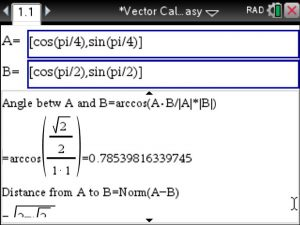## Find the angle (Theta) between the two vectors using Vector Calculus Made Easy for the Ti-Nspire CX CAS

Say we have to..

Find the angle (Theta) between the two vectors

U= cos(pi/4)i + sin(pi/4)j

V= cos(pi/2)i + sin(pi/2)j

When entering these 2 vectors using option 1 6 in Vector Calculus Made Easy we will not use the i-j notation and instead use vector /matrix notation as  shown in this image . The angle is derived using arccos(AB/|A|*|B|) and given in radian format.Note: We know that the vectors differ by an angle of pi/2-pi/4=pi/4 or .785  , which also equals 45 degrees.

Posted on Categories angle, calculus, vector